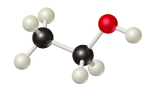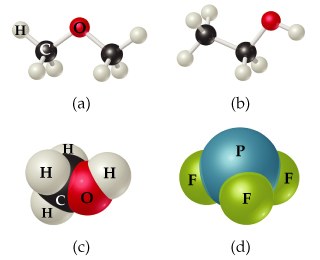# Problem: Write the molecular and structural formulas for the compounds represented by the molecular models in the figure .Write the molecular formula for the compound (b).

###### FREE Expert Solution

We are asked to write the molecular and structural formulas for the compound below:For this, we need to do the steps below:

• Step 1. Identify the elements represented by each ball in the model.
• Step 2. Write the structural formula of the compound.
• Step 3. Write the molecular formula of the compound.###### Problem Details

Write the molecular and structural formulas for the compounds represented by the molecular models in the figure.

Write the molecular formula for the compound (b).